# Calculating SLOPE in Excel

The SLOPE function returns the slope of the regression line created by known x's and know y's. It is a statistical function and used in various analysis projects including regression analysis.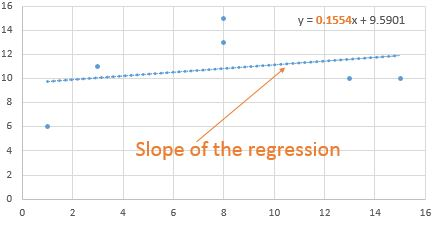What is the slope formula?

In mathematical terms, it is the number telling the steepness of the line. It is denoted by m. It can be visualized as a straight line that connects two points on the graph. A negative slope number tells us that the line is going downwards on the right.  A positive slope tells us that the line is going upward on the right of the graph. The mathematical formula for calculating the slope of known x's and know y's is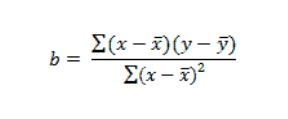Where x bar is the mean of the know x's and y bar is the mean of known y's.

Luckily, we don't have to calculate the slope manually. excel provides the SLOPE function to calculate the slope of a line. But to understand it, in the end, we will calculate the slope of the line manually in excel.

Syntax of SLOPE

 =SLOPE(known_ys,known_xs)

known_ys: Known the values of y.

known_xs: Known the values of x.

Note: The number of observations of x and y should be the same.

Let's see an example of how to find a Slope in excel.

Example: Find the slope of the regression line created by known ys and xs

Here I have a small data set to explain how to use the SLOPE function in excel. This data contains known xs in range A2:A7 and known ys are in range B2:B7. Note that they have the same number of observations (6).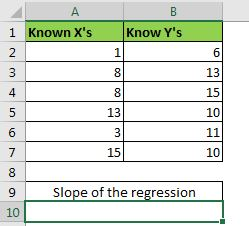In Cell A10 write this formula.

 =SLOPE(B2:B7,A2:A7)

We have a slope of data provided, as 0.155405405. This can be rounded up to 0.16.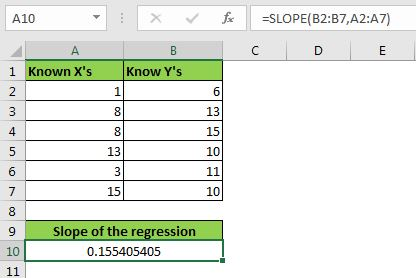Interpretation:

What does that mean for having a slope of 0.16? This means that for every 1 unit increase of x, y will increase with 0.16. You can also tell that the slope is going upwards on the right of the graph since it is positive.

How does it work?

To understand how we got our slope of the line we have to understand the mathematics of it and calculate it using basic mathematics in excel.

To calculate the slope of the data set manually, we need to translate the mathematical formula of the slope into excel formula.

First, we need the means of x and y. To calculate mean of xs and ys use the AVERAGE function.

 =AVERAGE(A2:A7)

In B10 write

 =AVERAGE(B2:B7)

Now we need to calculate the difference of means from each x and y. To do so write these formulas in C2 and D2.

 =A2-\$B\$9
 =B2-\$B\$10

Drag them down.

Now we need the product of these differences (see formula above).  Multiply D2 with C2 and drag it down.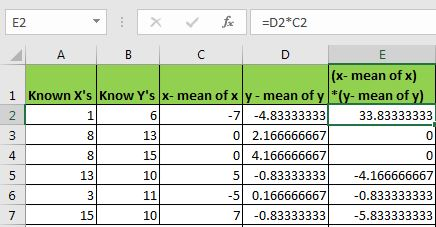Now get square of each x- mean of x (see mathematical formula above). In cell F2, write this formula and drag it down.

 =POWER(C2,2)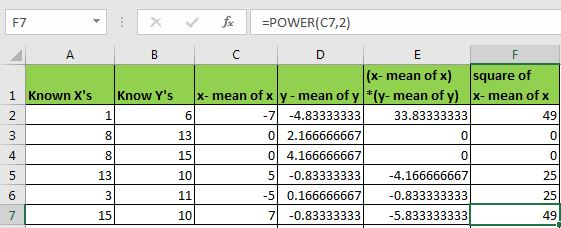Now sum the range E2:E7 and F2:F7 (following the formula)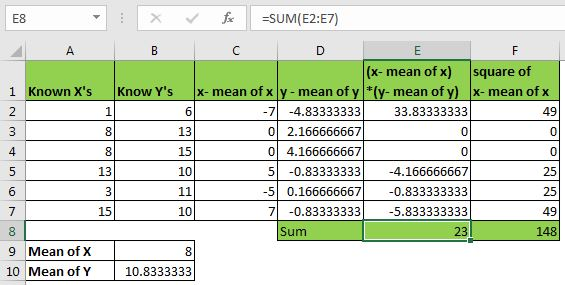We are all set to calculate the SLOPE of this data. Just divide the sum of  (x- mean of x) *(y- mean of y) by the sum of the square of x- mean of x. In excel words, divide E8 by F8

In cell A13, write this formula.

 =E8/F8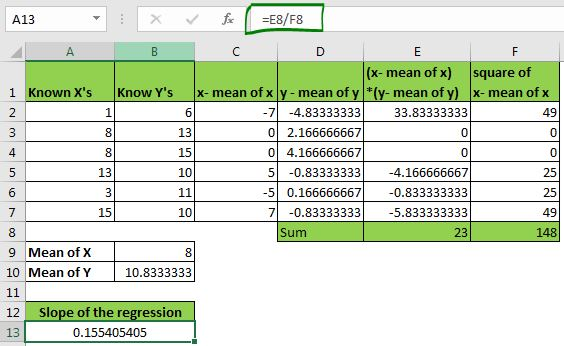We have aur slope value again. you can check it by using the SLOPE function. They are the same. The only difference was the exhausting process. However, this process helps you understand how the slope is calculated in the backend.

So yeah guys, this is how we use the SLOPE function of excel. I hope this was helpful. If you have any doubts or suggestions, write down it in the comments section below.

Related Data:

How to Use STDEV Function in Excel

How to Use STDEV.P Function in Excel

Regressions Analysis in Excel

How To Calculate Mean

Popular Articles:

The VLOOKUP Function in Excel

COUNTIF in Excel 2016

How to Use SUMIF Function in Excel

Terms and Conditions of use

The applications/code on this site are distributed as is and without warranties or liability. In no event shall the owner of the copyrights, or the authors of the applications/code be liable for any loss of profit, any problems or any damage resulting from the use or evaluation of the applications/code.